••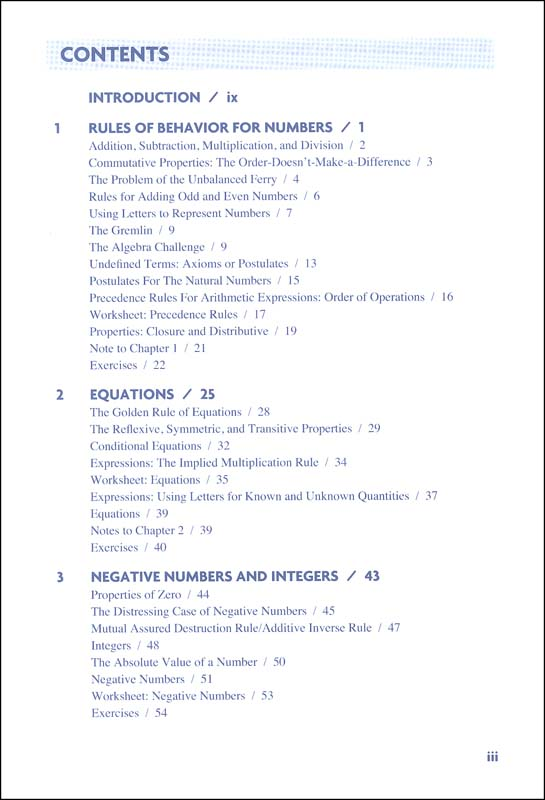•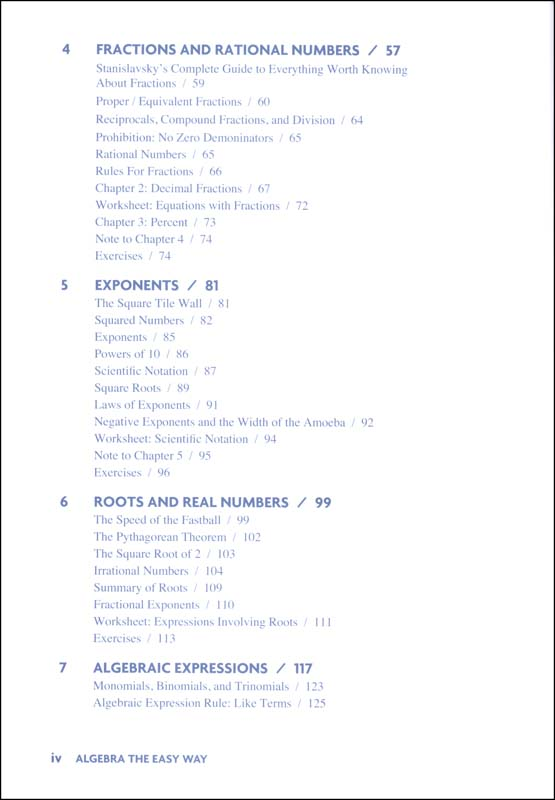•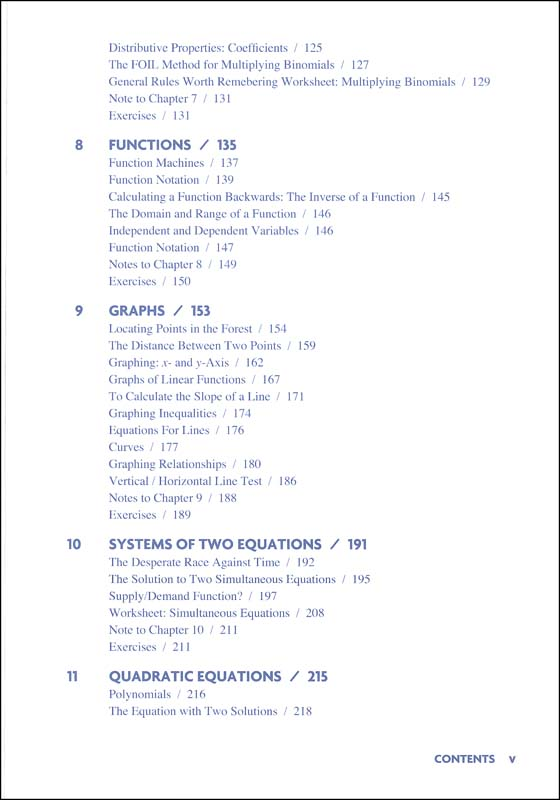•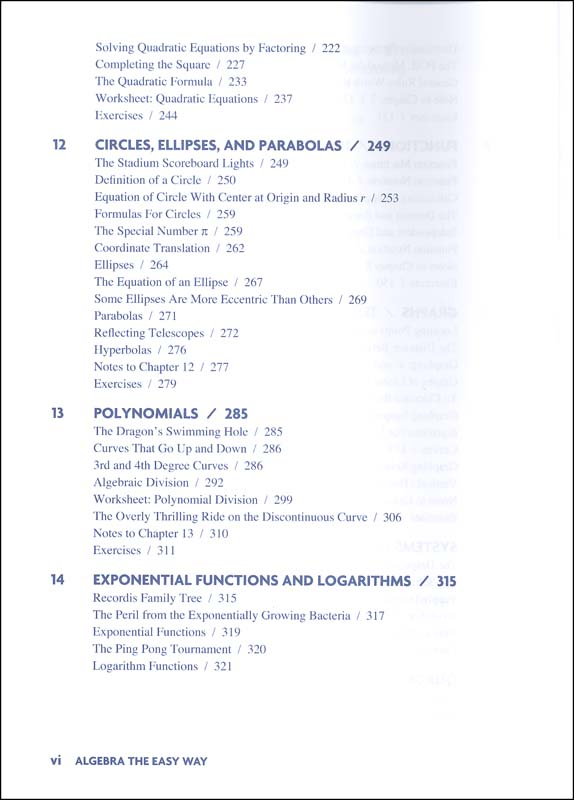•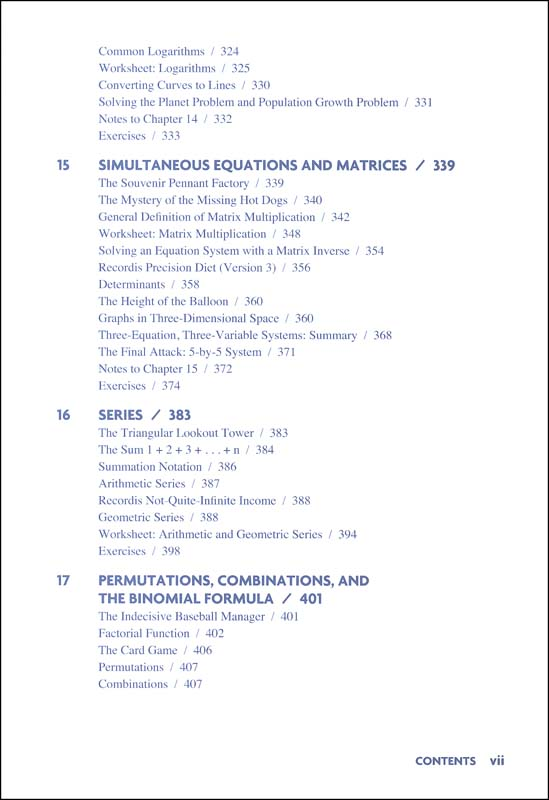•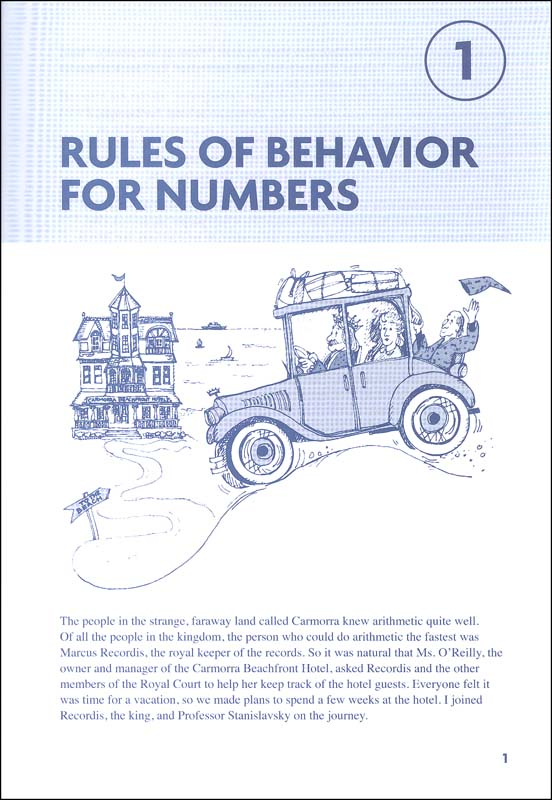•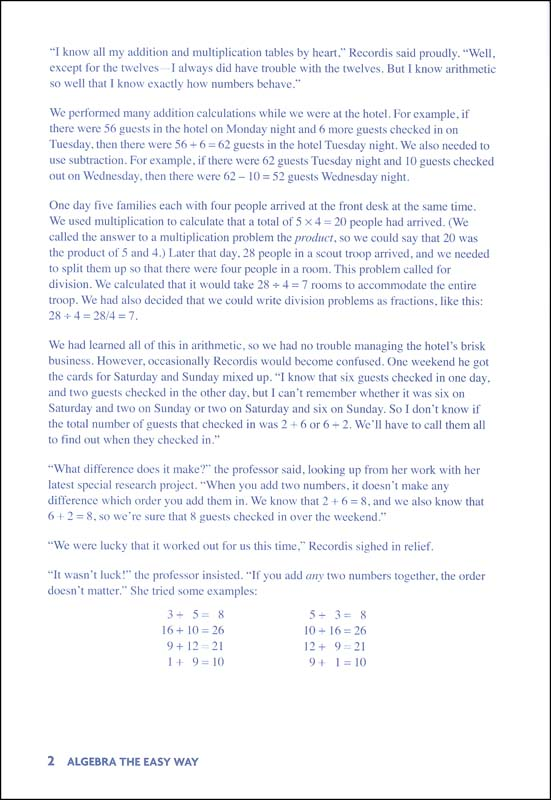•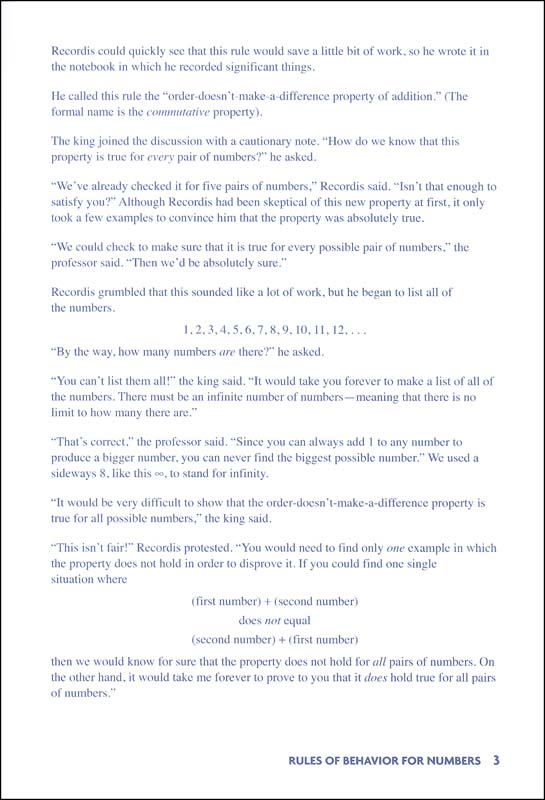•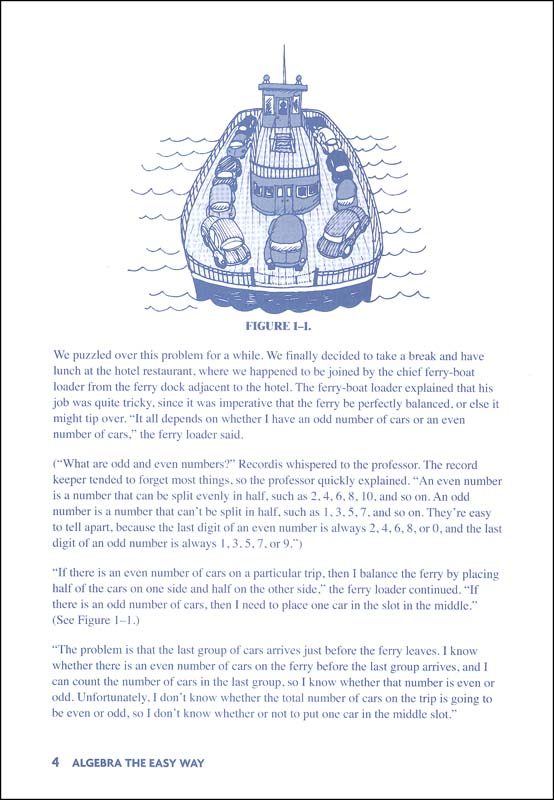•# Algebra: The Easy Way

# 005020

Our Price: \$11.95
Retail: \$16.99
Save: 29.66% (\$5.04)
3 In Stock.
Qty:
Qty:

Item #: 005020 9781438012131 9-12

#### Product Description:

Uses a storyline approach, putting skills in meaningful topics as concepts are "discovered" to solve real problems (albeit in an imaginary land). Covers: rules of behavior for numbers, equations, negative numbers and integers, fractions and rational numbers, exponents, roots and real numbers, algebraic expressions, functions, graphs, systems of two equations, quadratic equations, circles, ellipses, parabolas, polynomials, exponential functions and logarithms, simultaneous equations and matrices, series, permutations, combinations, the binomial formula, proofs by mathematical induction, and imaginary numbers.

#### Publisher Description:

A self-teaching guide for students, Algebra: The Easy Way provides easy-to-follow lessons with comprehensive review and practice.

This edition features a brand new design and new content structure with illustrations and practice questions.

An essential resource for:

• High school and college courses
• Virtual learning
• Learning pods
• Homeschooling

Algebra: The Easy Way covers:

• Numbers
• Equations
• Fractions and Rational Numbers
• Algebraic Expressions
• Graphs
• And more!

Category Description for E-Z Math:

This book has some overlapping content with Arithmetic the Easy Way. There's a short review of the four basic operations in chapter one, but then it jumps right into fractions, decimals, percents, beginning algebra, factoring, equations, geometry, tables and graphs, word problems, and probability and statistics. Explanations of concepts and terminology are clear and to the point. Algebraic processes, such as graphing a linear equation or solving a quadratic equation, are systematically broken down and presented as step-by-step procedures. Steps in worked examples are correspondingly numbered for clarity. Each chapter includes a pretest, a posttest, and numerous practice sets. The answer key also provides solutions for more difficult practice problems. 230 pgs. ~ Anh

Primary Subject
Mathematics
9
12
ISBN
9781438012131
Author
Douglas Downing Ph.D.
Format
Softcover Book
Brand Name
Barron's Educational Series
Weight
2.0 (lbs.)
Dimensions
10.0" x 7.81" x 1.25"
Browse 1 question Browse 1 question and 3 answers
Why did you choose this?
Rainbow Resource Center Store
My son is struggling with Algebra. So I'm looking for something to help him understand better.
on Oct 16, 2018
Just supplemental work for my freshman
on Sep 28, 2015
My son is struggling with Algebra. So I'm looking for something to help him understand better.
on Oct 16, 2018
I need it
on Aug 27, 2016
I need it
on Aug 27, 2016
5.0 / 5.0
1 Review
5 Stars
4 Stars
3 Stars
2 Stars
1 Star
1
0
0
0
0
Rated 5 out of 5
Great summer homework
The only thing, I would add more exercises at the end of every chapter.
June 29, 2018
Purchased
over 4 years ago

help desk software